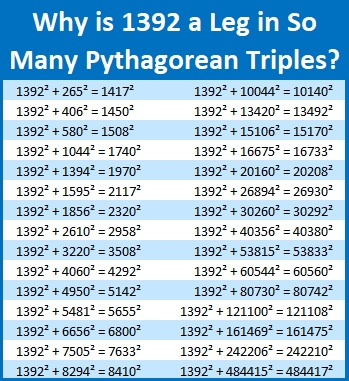# 1392 and Pythagorean Triples

1392 is the hypotenuse of ONE Pythagorean triple, 960-1008-1392.

However, 1392 is the leg of so many Pythagorean triples, that it is possible I haven’t listed them all in this graphic:Why is it the hypotenuse only once, but it is a leg so many times?

Because of its factors!

• 1392 is a composite number.
• Prime factorization: 1392 = 2 × 2 × 2 × 2 × 3 × 29, which can be written 1392 = 2⁴ × 3 × 29
• 1392 has at least one exponent greater than 1 in its prime factorization so √1392 can be simplified. Taking the factor pair from the factor pair table below with the largest square number factor, we get √1392 = (√16)(√87) = 4√87
• The exponents in the prime factorization are 4, 1 and 1. Adding one to each exponent and multiplying we get (4 + 1)(1 + 1)(1 + 1) = 5 × 2 × 2 = 20. Therefore 1392 has exactly 20 factors.
• The factors of 1392 are outlined with their factor pair partners in the graphic below.1392 has only one prime factor that leaves a remainder of one when it is divided by four. That factor is 29. It makes 960-1008-1392 simply (20-21-29) times 48. Easy Peasy.

ONE of the reasons it is a leg so many times is because several of its factors are in primitive Pythagorean triples, and multiplying those triples by that factor’s factor pair gives us a triple with 1392 as a leg:

• (3-4-5) times 464 is (1392-1856-2320)
• (3-4-5) times 348 is (1044-1392-1740)
• (8-15-17) times 174 is (1392-2610-2958)
• (5-12-13) times 116
• (12-35-37) times 116, and so on

Another reason is every Pythagorean triple can be written in this form 2ab, a²-b², a²+b², and 1392 = 2(696)(1) or 2(348)(2) or 2(232)(3) or 2(174)(4) and so on.

The last reason is that since 1392 has six factor pairs in which both factors are even, it can be written as a²-b²: (The average of the two numbers in the factor pair gives us the first number to be squared. Subtract the second number from it to get the second number to be squared.)

• 696 and 2 give us 349² – 347² = 1392
• 348 and 4 give us 176² – 172² = 1392
• 232 and 6 give us 119² – 113² = 1392
• 174 and 8 give us 91² – 83² = 1392
• 116 and 12 give us 64² – 52² = 1392
• 58 and 24 give us 41² – 17² = 1392

Some of the triples can be found by more than one of the processes listed above. It can be very confusing to keep track of them all. That is why I usually only write when a number is the hypotenuse of a triple and not when it is a leg.